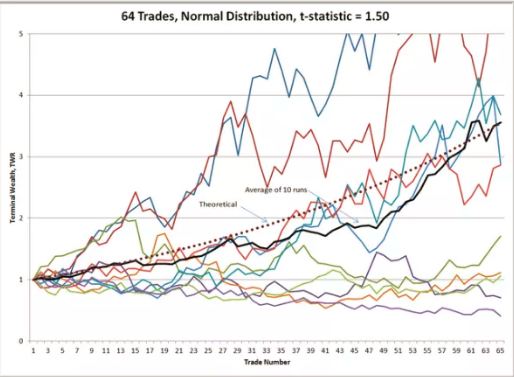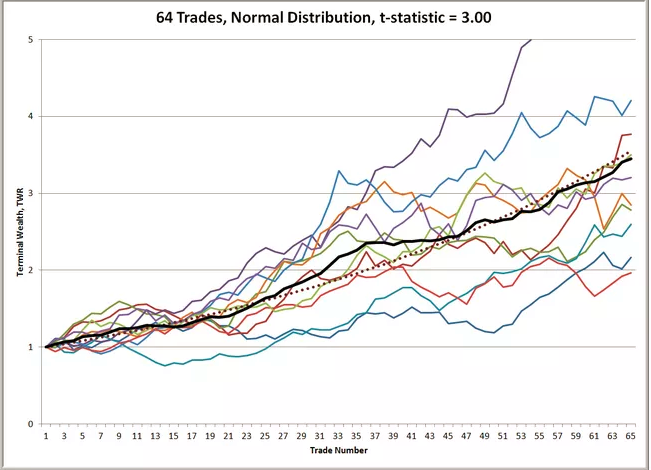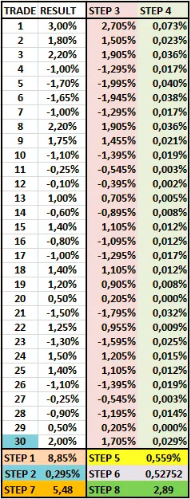# Traders Can Calculate Luck vs Skill Factor Using T-Statistic

You might be wondering if it is possible to know whether your trading performance is based on luck or skill? How do you know if your strategy is really doing that well or whether your trade setups were just a mere chance?

Interestingly, there is a method that allows traders to calculate and analyse their long-term performance called “T-statistic”. It shows whether your equity curve was based on a random distribution of results or on a clear path of growth.

This article explains what the T-statistic is, how it’s calculated, and how traders can use it for their analysis.

## What is T-Statistic Exactly?

The T-statistic is calculated based on the results of your trade setups. It could be the actual results from a live or demo account that you have achieved in the past weeks or months.

It can even be the results from your strategy testing but, of course, actual results will provide more accurate data because they include all market factors.

The T-statistic makes a mathematical calculation based on the results of each trade setup. It then calculates whether your setups have a wide or narrow expected distribution.

You might wonder, why does distribution matter? Here is how you can understand the difference:

• A wide distribution of results means that the results were more random. This, in turn, indicates that repeating the same results in the future is expected to be more difficult;
• A narrow distribution of results means that the results were less random. This, in turn, indicates that repeating the same results in the future is expected to be easier.

Traders can measure this distribution via the T-statistic, here is how:

• A higher T-statistic is better for traders as it indicates less randomness and more stable and similar results in the long-term;
• A lower T-statistic is worse for traders as it indicates more randomness and less stable and similar results in the long-term.## What is the T-Statistic and What is the Minimum Trade?

This week, I just measured the T-statistic value of setups from Nenad Kerkez and myself, plus calculated it for my own system. I will show you how to calculate the T-statistic in the next section.

It is important to know that level 2.0 is a key border. Anything above 2.0 is very good, whereas anything below 2.0 is weaker. Of course, the higher the better:

• 3.0 is better than 2.5;
• 1.8 (although below 2.0) is much better than 0.5.Keep in mind that traders need at least 40 to 50 trade setups to be able to calculate the results properly. Anything less than 40-50 setups makes no sense because a series of trades simply does not provide sufficient data.

That is why one loss or win is never (or should never be) a major problem for any trader (when proper risk management is applied). It’s about the long-term performance, not whether one trade wins or loses.

The minimum long-term perspective is 40-50 trades, but more is better. The more trades that are taken into account, the more reliable the T-statistic is.## How to Calculate T-Statistic

Here are the step-by-step instructions to calculate the T-statistic:

Step 1: choose a group of weeks, months, or trades you want to analyse. It’s good to have a sample size of minimum 40 trades.

Step 2: take all of your results and count the total of all setups. Use the percentage result of each setup and not the number of pips.

Step 3: divide the total result by the total number of trade setups to get the average result per trade setup.

Step 4: subtract the result of each setup with the average results per trade (see step 3). Let’s say your average is 0.35. Your results are +1, -0.5, etc. You will take each results (+1, -0.5) and subtract the average (0.35), which means: (+1 – 0.35 = 0.65) and (-0.5 – 0.35 = -0.85).

Step 5: multiply the result of each row of step 4 by step 4, which means: (0.65 * 0.65) and (-0.85 * -0.85).

Step 6: count the total of step 5.

Step 7: divide the average result per trade (step 2) by the total of step 6.

Step 8: calculate the square root of the total number of trade setups.

Step 9: multiply step 8 by step 7 = T-statistic.

Step 10: Get data on T-statistics You can open an account or demo account on our platform.

### Open an account now

It's free to open an account, takes less than five minutes, and there's no obligation to fund or trade.